Full Text:<2852>

CLC number: TU528.1

On-line Access: 2014-01-27

Revision Accepted: 2013-11-05

Crosschecked: 2014-01-14

Cited: 1

Clicked: 5134

Citations:  Bibtex RefMan EndNote GB/T7714

 Journal of Zhejiang University SCIENCE A 2014 Vol.15 No.2 P.138-148 http://doi.org/10.1631/jzus.A1300261Predicting unstable toughness of concrete based on initial toughness criterion*

 Author(s):  Long-bang Qing1,2, Wen-ling Tian1,2, Juan Wang3 Affiliation(s):  1. College of Civil Engineering, Hebei University of Technology, Tianjin 300401, China; more Corresponding email(s):   qlongbang@126.com Key Words:  Concrete, Fracture, Initial toughness, Unstable toughness, Wedge splitting tests Share this article to： More <<< Previous Article|Next Article >>>

Long-bang Qing, Wen-ling Tian, Juan Wang. Predicting unstable toughness of concrete based on initial toughness criterion[J]. Journal of Zhejiang University Science A, 2014, 15(2): 138-148.

@article{title="Predicting unstable toughness of concrete based on initial toughness criterion",
author="Long-bang Qing, Wen-ling Tian, Juan Wang",
journal="Journal of Zhejiang University Science A",
volume="15",
number="2",
pages="138-148",
year="2014",
publisher="Zhejiang University Press & Springer",
doi="10.1631/jzus.A1300261"
}

%0 Journal Article
%T Predicting unstable toughness of concrete based on initial toughness criterion
%A Long-bang Qing
%A Wen-ling Tian
%A Juan Wang
%J Journal of Zhejiang University SCIENCE A
%V 15
%N 2
%P 138-148
%@ 1673-565X
%D 2014
%I Zhejiang University Press & Springer
%DOI 10.1631/jzus.A1300261

TY - JOUR
T1 - Predicting unstable toughness of concrete based on initial toughness criterion
A1 - Long-bang Qing
A1 - Wen-ling Tian
A1 - Juan Wang
J0 - Journal of Zhejiang University Science A
VL - 15
IS - 2
SP - 138
EP - 148
%@ 1673-565X
Y1 - 2014
PB - Zhejiang University Press & Springer
ER -
DOI - 10.1631/jzus.A1300261

Abstract:
The fracture processes of concrete were described by a cohesive crack model based on initial toughness criterion. The corresponding analytical method to predict the instability state was proposed. In this model, the initial toughness was adopted as the crack propagation criterion and the weight function method was used to calculate the stress intensity factor and the crack opening displacement caused by the cohesive stress. The unstable toughness can be easily obtained using the proposed method without measuring parameters at the critical state that was necessary in traditional methods. The proposed method was verified by existing experimental data of wedge splitting specimens with different grades of concrete and the sensitivity of the results on the tensile softening curve was discussed. The results demonstrate that the proposed method can well predict the peak load, the critical effective crack length, and the unstable toughness of concrete specimens. Moreover, the calculated unstable toughness is not sensitive to the tensile softening curve.

## 1.  Introduction

A large number of experimental studies on concrete fracture have shown that an obvious fracture process zone (FPZ) exists at the crack tip (Bazant and Planas, ). The traditional linear elastic fracture mechanics (LEFM), is no longer applicable to the analysis of the concrete fracture process (Jenq and Shah, ) when the size of FPZ is comparable to the structure. To date, numerous nonlinear models for concrete fracture have been developed from different perspectives and based on various assumptions. The primary models and their characteristics are briefly summarized herein.

The fictitious crack model (FCM) (Hillerborg et al., ) and crack band model (CBM) (Bazant and Oh, ) both considering the softening relation in the FPZ, were primarily solved numerically. The size effect law (SEL) developed by Bazant () analyzes the size effect of the fracture by comparing nominal stress in the failure of structures with different sizes. The effective crack model (ECM) (Karihaloo and Nallathambi, ), known as the equivalent LEFM, has an explicit analytical solution. The two-parameter fracture model (TPFM) proposed by Jenq and Shah () was based on the assumption that crack propagation starts when the stress intensity factor and crack tip opening displacement (CTOD) reach their critical values. The critical stress intensity factor in both ECM and TPFM was calculated from the peak load P max and critical effective crack length a c using a formula developed in LEFM.

Three different stages in the concrete fracture processes have been identified, namely, crack initiation, stable crack propagation, and unstable crack propagation (Xu and Reinhardt, ). To depict the three stages of crack propagation without compromising simplicity, Xu and Reinhardt () developed the double-K model, which employed the initial toughness and the unstable toughness as control parameters of the two important instantaneous states of crack initiation and instability, respectively. Macroscopic damage in concrete is believed to be initiated once the stress intensity factor at the crack tip reaches (Zhang and Xu, ). Crack instability is the catastrophic point between the stable and unstable crack propagation stages and is significant in investigating the mechanisms of concrete fracture. The double-K model has been playing an important role in engineering practice. For instance, and have been used in safety warning systems (DL/T 5332-2005, ) and in evaluating the performance of concrete structures with cracks.

The initial toughness can be easily obtained using formulae of the initial cracking load and initial crack length based on LEFM (Xu and Reinhardt, ). Several measurement methods for the initial cracking load, such as tests using photo-elastic coating, laser speckle (Xu and Reinhardt, ) and strain gauge (Zhang and Xu, ), and analytical methods have been formulated. Xu and Reinhardt (; ; ) proposed the double-K method and developed a simplified method later. Kumar and Barai () developed a weight function method. The comparison of the above analytical methods can be found in (Zhang and Xu, ). Recently, Qing and Li () proposed a theoretical method to obtain based on experimental peak load. Alternatively, as an easy method, Reinhardt and Xu () and Zhang et al. () determined the initial cracking load by the transition point that separates the linear and nonlinear segments of the load P-CMOD (crack mouth opening displacement) curve.

Nevertheless, predicting the unstable toughness is difficult without making any assumption. In the experimental aspect, can be calculated by the peak load P max and the critical effective crack length a c, which were mainly measured at the peak load state through the three point bending (TPB) specimens or wedge splitting (WS) specimens (DL/T 5332-2005, ). However, the critical effective crack length a c was usually difficult to measure accurately. In traditional methods, a c was calculated by critical crack mouth opening displacement CMODc through empirical formulae (Xu and Reinhardt, ; ; Kumar and Barai, ; Zhang and Xu, ). Furthermore, in the numerical aspect, a similar step-by-step procedure must be followed until the maximum load was reached (Wu et al. ; Dong et al., ). Therefore, it is of significance to establish a simple theoretical method for predicting the unstable toughness .

Based on the analysis of concrete fracture mechanisms, the current study attempts to develop an easy-to-use theoretical method for predicting the unstable toughness . In this method, the initial toughness was adopted as the control parameter of crack propagation and a weight function method was used to calculate the stress intensity factor and the crack opening displacement caused by the cohesive stress. Then the proposed method was verified using experimental data on wedge splitting specimens. The sensitivity of the results to the tensile softening curve was discussed.

## 2.  Model development

### 2.1.  Fracture processes of concrete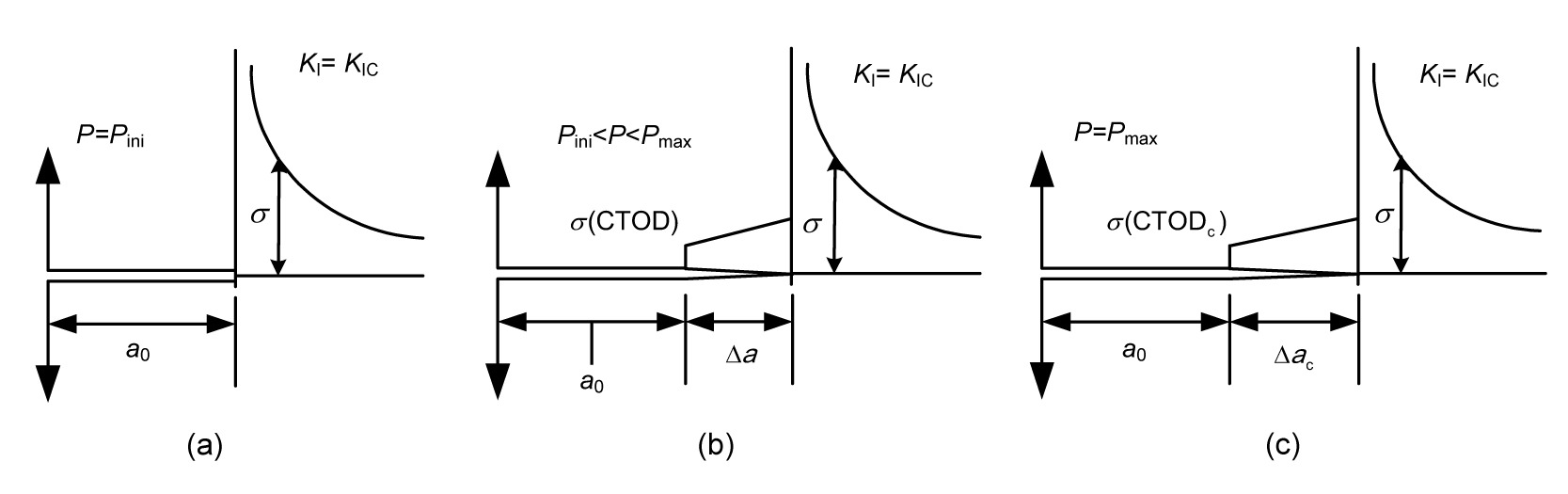Fig.1
Three stages of the concrete fracture processes
(a) Crack initiation; (b) Stable crack growth; (c) Unstable fracture

A typical P-a/D (a is the effective crack length, and D is the specimen height) curve is shown in Fig. 2 (Reinhardt and Xu, ; Kumar and Barai, ; Qing and Li, ). When P reaches the initial cracking load P ini, crack begins to grow and it gradually and nonlinearly increases with a. When P reaches the peak load P max, a=a c. Then P gradually decreases with a. The derivation of P to a at P=P max can be assumed to be continuous (Qing and Li, ). The maximum theory can also be adopted to predict the crack instability.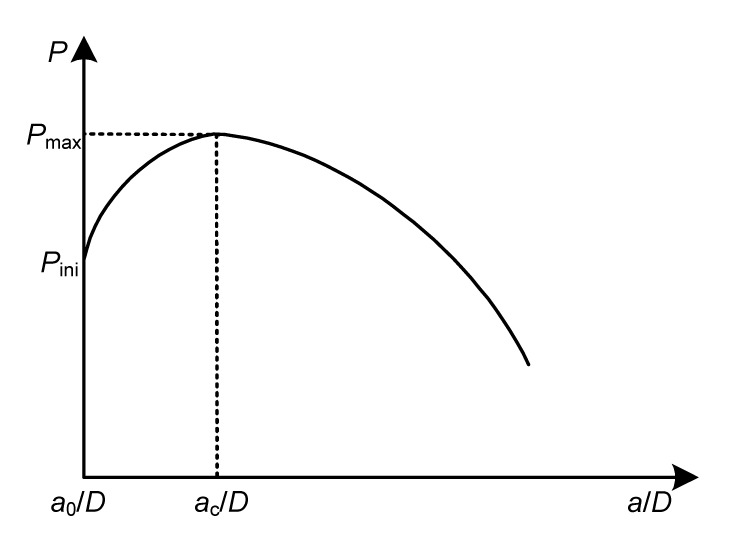Fig.2
A typical P-a/D curve of concrete fracture (Qing and Li, )

### 2.2.  Criterion for concrete fracture

As discussed above, a crack is initiated in concrete once the external load reaches the crack load P ini or the stress intensity factor increases to the initial toughness . Thus, can be regarded as the toughness of the structural material to crack growth attributable to external forces. In the present study, the following criterion for crack initiation and propagation was employed (Xu and Reinhardt, ; Wu et al., ; Dong et al., ): , where K I is the stress intensity factor at the tip of the effective crack tip in a mode I fracture.

Before crack initiation, LEFM can be applied, and K I can be calculated by , where K I P is the stress intensity factor attributable to the external load in a mode I fracture.

After the crack initiation, K I can be expressed by the superposition scheme: , where K I C is the stress intensity factor attributable to cohesive stress.

### 2.3.  Cohesive stress distribution

It is assumed that the cohesive stress distribution on the FPZ can be expressed by Eq. (3) (Li et al., ). The cohesive stress at the tip of the effective crack equals the tensile strength. The cohesive stress at the tip of the initial crack and the crack tip opening displacement CTOD satisfy the tensile softening curve. , where σ s is the stress on the initial crack tip, f t is the tensile strength, and m is the cohesion distribution index.

According to Eq. (3), the distribution of cohesive stress changes with the index m. When m=1, the cohesive stress distribution is linear. Eq. (3) is similar to the cohesive force distribution adopted by Reinhardt (). The difference is that the cohesive stress at the initial crack tip is not zero in the present model. Based on the theoretical model, the corresponding analytical approach is developed in the following section.

## 3.  Analytical method for fracture

Take the WS specimen for example, the configuration of the WS specimen is shown in Fig. 3 (DL/T 5332-2005, ).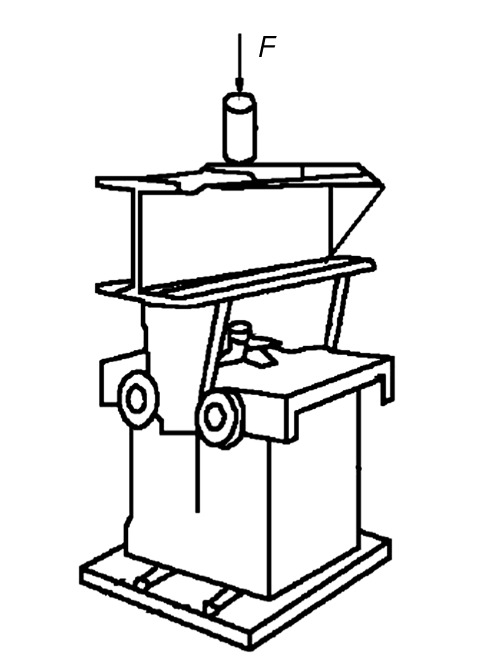Fig.3
Test set-up of WS specimen (DL/T 5332-2005, )

K I P can be expressed by (Xu and Reinhardt, ): , where α=a/D, and k(α) is a geometric factor, which can be calculated by (DL/T 5332-2005, ): . Eq. (5) is valid for 0.2≤α≤0.8 with 2% accuracy (Xu and Reinhardt, ).

To obtain the value of K I C, a distribution of cohesive stress is assumed on the fictitious crack in an infinite strip (Jenq and Shah, ). Previous studies have shown that the descending segment of the P-CMOD curve is affected by the cohesive stress distribution, while the peak load and critical crack mouth opening displacement are almost not affected (Li et al., ). Therefore, to simplify the analysis of the fracture processes, a linear cohesive stress assumption (m=1) was adopted for the propagation state of concrete cracks in the current study. The cohesive stress distribution is shown in Fig. 4, where σ s(CTOD) is the function of CTOD.

The weight function method proposed by Kumar and Barai () was adopted in this study to calculate KI C: , where g(a) can be expressed using the following fourth-order weight function: , where A 1=σ s(CTOD), A 2=[f tσ(CTOD)]/(aa 0), s=1−a 0/a, M 1, M 2, and M 3 can be expressed by α (Kumar and Barai, ).

The CTOD and σs(CTOD) values are required to calculate K I C using Eqs. (6) and (7), where σs(CTOD) can be expressed by (Reinhardt et al., ): , where c 1, c 2, and w 0 are parameters.

CTOD in Eq. (8) needs to be calculated by adding up two displacements caused by the external load P and cohesive stress. Using Paris’ displacement formula (Tada et al., ; Mai, ), CTOD can be expressed by , where E is the elasticity modules, ξ is the integration variable, m(x, a) can be expressed using the following fourth-order weight function (Kumar and Barai, ): .

Thus, using Eqs. (7)–(9), g(a) can be derived, and KI C can be obtained using Eq. (6). Then, substituting Eq. (4) for K I P and Eq. (6) for K I C into Eq. (2b) leads to: , where

Eq. (11) obviously shows that the external load P can be explicitly expressed as a function of the effective crack length a. The derivation of this equation with respect to a at the moment of the crack instability can be expressed by the following partial differential equation: , and is calculated by , where

g′(a) can be expressed as , where

k′(α) can be expressed as .

If the initial toughness is given, the critical effective crack length a c can be calculated using Eq. (12) by iterating method. Then, the critical crack tip opening displacement CTODc and the peak load P max can be obtained by substituting a=a c into Eq. (9) and Eq. (11), respectively. The unstable toughness can be calculated by substituting a=a c and P=P max into Eq. (4). Hence, an analytical approach for calculating was developed.

## 4.  Results and discussion

### 4.1.  Verification of the analytical method

In this section, the proposed method for concrete fracture was verified using the data from Xu et al. ()’s experiments on WS specimens with different maximum aggregate sizes. The parameters of the specimens are shown in Table 1. In the experiments, the crack initiation load P ini was measured by resistance strain gauges and the initial toughness was obtained by the LEFM formula.

#### Table 1

Parameters of the wedge splitting specimens
 Specimen No. Specimen size, 2H×D×B (mm) Maximum aggregate size (mm) a 0 (mm) E (GPa) Compressive strength, f c (MPa) Tensile strength, f t (MPa) WS13 300×300×200 20 150 33.4 34.2 2.76 WS14 600×600×200 20 300 33.4 34.2 2.76 WS15 800×800×200 20 400 33.4 34.2 2.76 WS16 1000×1000×200 20 500 33.4 34.2 2.76 WS17 1200×1200×200 20 600 33.4 34.2 2.76 WS32 300×300×200 40 150 29.1 34.3 3.04 WS22 600×600×200 40 300 29.1 34.3 3.04 WS34 800×800×200 40 400 29.1 34.3 3.04 WS35 1000×1000×200 40 500 29.1 34.3 3.04 WS23 600×600×250 80 300 29.1 34.3 3.04 WS24 800×800×250 80 400 29.1 34.3 3.04 WS25 1000×1000×250 80 500 29.1 34.3 3.04 WS26 1200×1200×250 80 600 29.1 34.3 3.04

According to the experimental conditions, the parameters in Eq. (8) for the proposed method were taken as follows: c 1=3, c 2=6.93, and w 0=160 μm (Reinhardt et al., ).

The calculated and measured values of the peak load P max are compared in Tables 24. It can be seen from the comparison that the values of the peak load P max calculated by the proposed method are generally in good agreement with the experimental values for different maximum aggregate sizes of concrete specimens. It can be concluded that the method is effective for predicting the instability of concrete.

#### Table 2

Comparison of the predicted and measured results (maximum aggregate size: 20 mm)
 Specimen No. Specimen size, 2H×D×B (mm) a 0 (mm) P ini (kN) Predicted a c/D Predicted P max (kN) Experimental P max (kN) Predicted (1) Experimental (2) (1)/(2) WS13-1 300×300×200 150 7.181 0.620 11.668 12.173 1.637 1.690 0.969 WS13-2 300×300×200 150 10.916 0.567 14.156 12.801 1.642 1.958 0.838 WS13-4 300×300×200 150 7.909 0.607 12.095 11.492 1.614 1.759 0.918 Mean 8.669 0.598 12.639 12.155 1.631 1.802 0.908 WS14-1 600×600×200 300 19.308 0.569 25.387 25.550 2.098 2.224 0.943 WS14-2 600×600×200 300 18.484 0.583 24.787 22.667 2.148 2.462 0.872 WS14-4 600×600×200 300 18.000 0.583 24.437 23.408 2.117 2.004 1.057 Mean 18.597 0.578 24.870 23.875 2.121 2.230 0.957 WS15-1 800×800×200 400 23.788 0.597 32.848 30.758 2.596 2.191 1.185 WS15-2 800×800×200 400 24.546 0.584 33.387 31.136 2.511 2.135 1.176 WS15-3 800×800×200 400 17.403 0.639 28.776 29.351 2.667 2.102 1.269 Mean 21.912 0.607 31.670 30.415 2.591 2.143 1.210 WS16-1 1000×1000×200 500 32.495 0.570 42.677 42.137 2.741 2.974 0.922 WS16-2 1000×1000×200 500 30.478 0.584 41.205 39.000 2.777 2.851 0.974 WS16-3 1000×1000×200 500 24.235 0.612 36.929 31.494 2.753 2.250 1.224 Mean 29.069 0.589 40.270 37.544 2.757 2.692 1.040 WS17-1 1200×1200×200 600 33.368 0.584 46.734 46.326 2.878 3.231 0.891 WS17-2 1200×1200×200 600 36.699 0.584 49.142 55.183 3.026 3.028 0.999 WS17-3 1200×1200×200 600 40.045 0.570 51.689 50.355 3.033 3.112 0.975 Mean 36.704 0.579 49.188 50.621 2.979 3.124 0.955

#### Table 3

Comparison of the predicted and measured results (maximum aggregate size: 40 mm)
 Specimen No. Specimen size, 2H×D×B (mm) a 0 (mm) P ini (kN) Predicted a c/D Predicted P max (kN) Experimental P max (kN) Predicted (1) Experimental (2) (1)/(2) WS32-1 300×300×200 150 8.234 0.593 12.237 11.221 1.556 1.524 1.021 WS32-2 300×300×200 150 8.848 0.580 11.384 9.433 1.381 1.232 1.121 WS32-3 300×300×200 150 8.431 0.593 12.366 10.727 1.572 1.371 1.147 Mean 8.504 0.589 11.996 10.460 1.503 1.376 1.096 WS33-2 600×600×200 300 18.973 0.569 25.017 24.511 2.068 1.957 1.057 WS33-3 600×600×200 300 18.588 0.569 24.725 21.956 2.044 2.132 0.959 WS33-4 600×600×200 300 16.091 0.583 22.912 21.242 1.985 2.006 0.990 Mean 17.884 0.574 24.218 22.570 2.032 2.032 1.002 WS34-1 800×800×200 400 22.306 0.584 30.683 27.349 2.308 2.016 1.145 WS34-2 800×800×200 400 18.766 0.597 28.215 27.049 2.230 2.106 1.059 WS34-4 800×800×200 400 26.711 0.570 34.006 32.000 2.439 2.713 0.899 Mean 22.594 0.584 30.968 28.799 2.326 2.278 1.034 WS35-2 1000×1000×200 500 21.337 0.598 33.364 29.866 2.363 2.071 1.141 WS35-3 1000×1000×200 500 19.284 0.612 32.076 25.634 2.391 1.884 1.269 WS35-4 1000×1000×200 500 21.837 0.598 33.698 32.700 2.386 2.336 1.021 Mean 20.819 0.603 33.046 29.400 2.380 2.097 1.144

#### Table 4

Comparison of the predicted and measured results (maximum aggregate size: 80 mm)
 Specimen No. Specimen size, 2H×D×B (mm) a 0 (mm) P ini (kN) Predicted a c/D Predicted P max (kN) Experimental P max (kN) Predicted (1) Experimental (2) (1)/(2) WS23-2 600×600×250 300 16.525 0.638 27.138 23.407 2.312 2.465 0.938 WS23-4 600×600×250 300 17.451 0.624 27.675 24.340 2.233 2.260 0.988 Mean 16.988 0.631 27.407 23.874 2.273 2.363 0.963 WS24-1 800×800×250 400 29.425 0.584 40.357 36.833 2.429 2.867 0.847 WS24-3 800×800×250 400 31.575 0.584 41.924 35.859 2.523 2.385 1.058 WS24-4 800×800×250 400 19.594 0.639 34.058 32.178 2.525 2.766 0.913 Mean 26.865 0.602 38.780 34.957 2.492 2.673 0.939 WS25-1 1000×1000×250 500 25.000 0.626 42.102 43.711 2.649 2.814 0.941 WS25-2 1000×1000×250 500 34.093 0.584 48.006 45.163 2.588 3.043 0.850 WS25-4 1000×1000×250 500 20.590 0.640 39.637 39.194 2.636 2.308 1.142 Mean 26.561 0.617 43.248 42.689 2.624 2.722 0.978 WS26-1 1200×1200×250 600 45.168 0.570 60.206 54.000 2.826 2.931 0.964 WS26-2 1200×1200×250 600 37.663 0.598 54.773 47.340 2.836 3.326 0.853 Mean 41.416 0.584 57.490 50.670 2.831 3.129 0.909

Moreover, the values of unstable toughness are also shown in Tables 24. The calculated values of by the proposed method generally agree well with those by experimental method. Some of the calculated results are slightly different from those obtained by the experimental method, for example, the ratio of the predicted result to experimental result of WS15 is 1.21. According to Eq. (4), the discrepancy in the unstable toughness results from the critical relative effective crack length a c/D. The primary reason is that, as mentioned above, an empirical formula was adopted in the experimental method to calculate the critical effective length a c. The proposed method, differing from the experimental method, is capable of considering the effects of both external load and cohesive force when calculating a c.

### 4.2.  Effect of tensile softening curve

To study the sensitivity of the method to the shape of the tensile softening curve, two groups of parameters characterizing different tensile softening curves were used to predict fracture using the proposed theoretical method.

The parameters of first group were c 1=3, c 2=6.93, and w 0=160 μm, which were adopted in the last section. For comparison, the second set of the softening curve parameters was taken as as c 1=1.5, c 2=6.3, and w 0=90 μm. The tensile softening curves represented by these two sets of parameters are shown in Fig. 5.

With these different parameters, the values of P max, a c/D, and were calculated using the proposed method, and the results are shown in Figs. 68. As can be seen from Figs. 68, the calculated P max, a c/D, and using the first group of parameters are slightly different from those of the other one. Generally, this disparity in the calculated results using these two sets of parameters is considered to be insignificant in view of the great difference between the two tensile softening curves. For example, the fracture energy G F (area under the tensile softening curve) corresponding to the first softening curve is about twice that for the second one.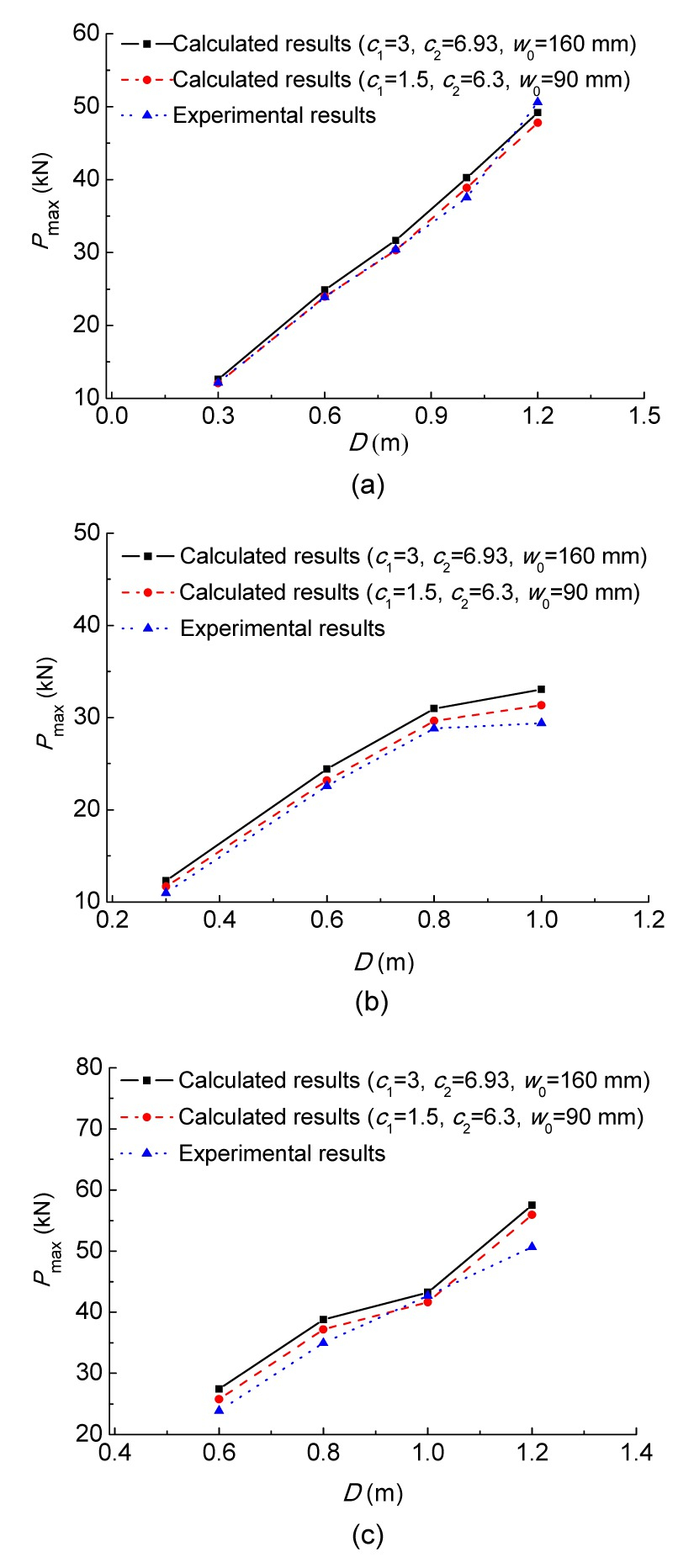Fig.6
Average values of P max for specimens with different maximum aggregate sizes of 20 mm (a), 40 mm (b), and 80 mm (c)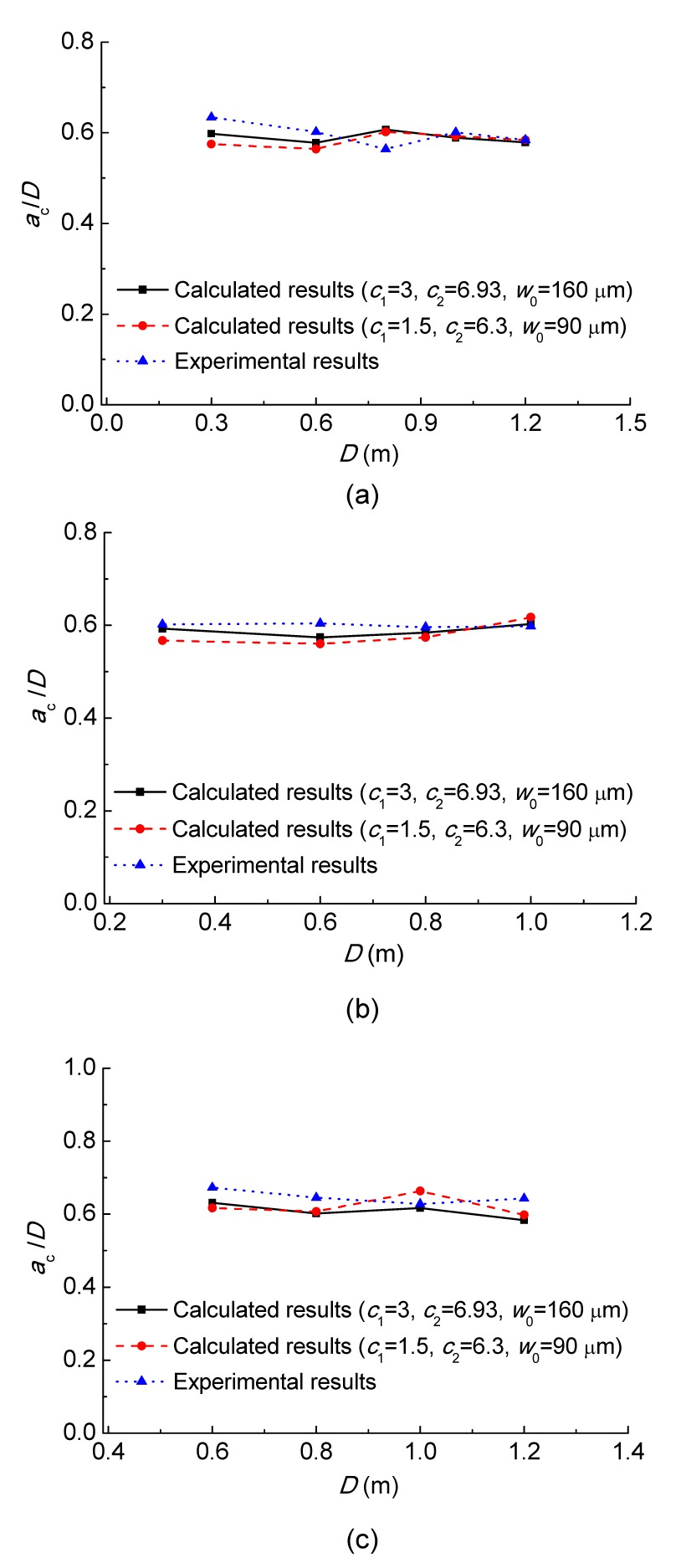Fig.7
Average values of a c/D for specimens with different maximum aggregate sizes of 20 mm (a), 40 mm (b), and 80 mm (c)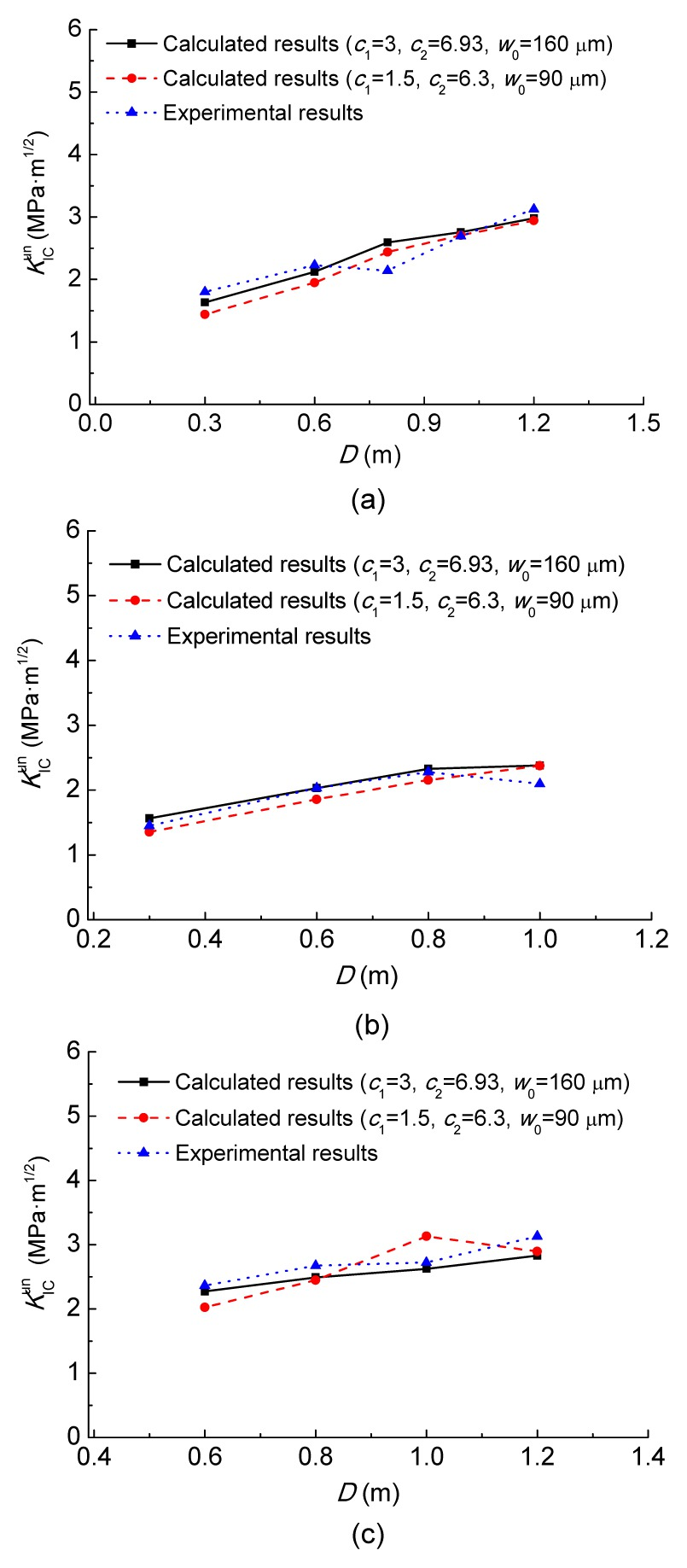Fig.8
Average values of for specimens with different maximum aggregate sizes of 20 mm (a), 40 mm (b), and 80 mm (c)

## 5.  Conclusions

In this study, the instability state of fracture in concrete was predicted by a theoretical model which adopted the initial toughness as the crack propagation criterion, and used the weight function method to calculate the stress intensity factor and the crack opening displacement caused by the cohesive stress. The applicability of the proposed method was verified by experimental data obtained on WS specimens, and the parameters at the peak load state, such as the peak load P max, critical effective crack length a c and unstable toughness , were calculated using the proposed method. The good agreement between the calculated results and the experimental results demonstrates that the proposed method can accurately predict the unstable toughness . In addition, the sensitivity of the results to the tensile softening curve was discussed. The results showed that the proposed method for fracture is not sensitive to the tensile softening curve, which verifies the reasonability of the proposed method. Future studies can be conducted from many aspects, such as the investigation of measurement methods for the effective crack length a c.

* Project supported by the National Natural Science Foundation of China (Nos. 51309073 and 51309203), the Specialized Research Fund for the Doctoral Program of Higher Education (No. 20131317120012), and the Open Research Fund Program of State Key Laboratory of Hydroscience and Engineering (No. sklhse-2014-C-02), China

## ReferencesBazant, Z.P., 1984. Size effect in blunt fracture: concrete, rock, metal. Journal of Engineering Mechanics, 110(4):518-535.Bazant, Z.P., Oh, B.H., 1983. Crack band theory for fracture of concrete. Material and Structure, 16(3):155-177.Bazant, Z.P., Planas, J., 1998.  Fracture and Size Effect in Concrete and Other Quasibrittle Materials. CRC Press,Boca Raton :DL/T 5332-2005, 2006.  Specification for Fracture Test of Hydraulic Concrete. (in Chinese), China Electric Power Press,Beijing, China :Dong, W., Wu, Z.M., Zhou, X.M., 2013. Calculating crack extension resistance of concrete based on a new crack propagation criterion. Construction and Building Materials, 38:879-889.Elices, M., Planas, J., 1991. Material models.  Fracture Mechanics of Concrete Structures from Theory to Applications. Report of RILEM Technical Committee 90-FMT. Chapman & Hall,London :16-65.Hillerborg, A., Modeer, M., Petersson, P.E., 1976. Analysis of crack formation crack growth in concrete by means of fracture mechanics and finite elements. Cement and Concrete Research, 6(6):773-782.Jenq, Y.S., Shah, S.P., 1985. Two parameter fracture model for concrete. Journal of Engineering Mechanics, 111(10):1227-1241.Jenq, Y.S., Shah, S.P., 1985. A fracture toughness criterion for concrete. Engineering Fracture Mechanics, 21(5):1055-1069.Karihaloo, B.L., Nallathambi, P., 1990. Effective cracks model for the determination of fracture toughness (KICS) of concrete. Engineering Fracture Mechanics, 35(4-5):637-645.Kumar, S., Barai, S.V., 2008. Influence of specimen geometry and size-effect on the KR -curve based on the cohesive stress in concrete. International Journal of Fracture, 152(2):127-148.Kumar, S., Barai, S.V., 2009. Determining double-K fracture parameters of concrete for compact tension and wedge splitting tests using weight function. Engineering Fracture Mechanics, 76(7):935-948.Li, Q.B., Qing, L.B., Guan, J.F., 2012. Analysis of the whole fracture process of concrete considering effects of cohesive distribution. Journal of Hydraulic Engineering, (in Chinese),43(Sup.):31-36.Mai, Y.W., 2002. Cohesive zone and crack-resistance (R)-curve of cementitious materials and their fibre-reinforced composites. Engineering Fracture Mechanics, 69(2):219-234.Qing, L.B., Li, Q.B., 2013. A theoretical method for determining initiation toughness based on experimental peak load. Engineering Fracture Mechanics, 99:295-305.Reinhardt, H.W., 1985. Plain concrete modeled as an elastic strain-softening material at fracture. Engineering Fracture Mechanics, 22(5):787-796.Reinhardt, H.W., Xu, S.L., 1999. Crack extension resistance based on the cohesive force in concrete. Engineering Fracture Mechanics, 64(5):563-587.Reinhardt, H.W., Cornelissen, H.A.W., Hordijk, D.A., 1986. Tensile tests and failure analysis of concrete. Journal of Structural Engineering, 112(11):2462-2477.Tada, H., Paris, P.C., Irwin, G., 2000. The Stress Analysis of Cracks Handbook, , New York, :Wu, Z.M., Dong, W., Liu, K., Yang, S.T., 2007. Mode I crack propagation criterion of concrete and numerical simulation on complete process of cracking. Journal of Hydraulic Engineering, (in Chinese),38:46-52.Xu, S.L., Reinhardt, H.W., 1999. Determination of double-K criterion for crack propagation in quasi-brittle fracture, Part I: experimental investigation of crack propagation. International Journal of Fracture, 98(2):111-149.Xu, S.L., Reinhardt, H.W., 1999. Determination of double-K criterion for crack propagation in quasi-brittle fracture, Part II: analytical evaluating and practical measuring methods for three-point bending notched beams. International Journal of Fracture, 98(2):151-177.Xu, S.L., Reinhardt, H.W., 1999. Determination of double-K criterion for crack propagation in quasi-brittle fracture, Part III: compact tension specimens and wedge splitting specimens. International Journal of Fracture, 98(2):179-193.Xu, S.L., Reinhardt, H.W., 2000. A simplified method for determining double-K fracture parameters for three-point bending tests. International Journal of Fracture, 104(2):181-209.Xu, S.L., Zhou, H.G., Gao, H.B., Zhao, S.Y., 2006. An experimental study on double-K fracture parameters of concrete for dam construction with various grading aggregates. China Civil Engineering Journal, 39(11):50-62.Zhang, J., Leung, K.Y., Xu, S.L., 2010. Evaluation of fracture parameters of concrete from bending test using inverse analysis approach. Materials and Structure, 43(6):857-875.Zhang, X.F., Xu, S.L., 2011. A comparative study on five approaches to evaluate double-K fracture toughness parameters of concrete and size effect analysis. Engineering Fracture Mechanics, 78(10):2115-2138.